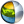Cg 3.1 Toolkit Documentation Cg / Standard Library / lit

## Name

lit - computes lighting coefficients for ambient, diffuse, and specular lighting contributions

## Synopsis

```float4 lit(float NdotL, float NdotH, float m);

half4 lit(half NdotL, half NdotH, half m);

fixed4 lit(fixed NdotL, fixed NdotH, fixed m);
```

## Parameters

NdotL
The dot product of a normalized surface normal and a normalized light vector.
NdotH
The dot product of a normalized surface normal and a normalized half-angle vector (for Blinn-style specular) where the half-angle vector is the sum of the normalized view vector and normalized light vector. Alternatively, the dot product of a normlized light vector and a normalized view vector reflected by a normalized surface normal could be used (for Phong-style specular).
m
A specular exponent, typically described as a measure of shininess. The larger the exponent, the shinier the specular highlight, the smaller the exponent, the duller the specular highlight.

## Description

The lit function is a helper function useful to compute lighting coefficients for ambient, diffuse, and specular lighting contributions. The function efficiently maps to a native instruction for most GPUs.

lit returns a 4-component vector arranged as follows:

x
The ambient coefficient that is always 1.0.
y
The diffuse coefficient that is zero if NdotL is less than zero, and NdotL otherwise.
z
The specular coefficient that is zero if either NdotL or NdotH are less than zero, and otherwise NdotH raised to the power m.
w
Always 1.0.

## Reference Implementation

lit accepting <float> parameters could be implemented this way:

```float4 lit(float NdotL, float NdotH, float m)
{
float specular = (NdotL > 0) ? pow(max(0.0, NdotH), m);
return float4(1.0, max(0.0, NdotL), specular, 1.0);
}
```

## Profile Support

lit is supported in all profiles.| Cg Toolkit | Download | Release Archive | Profiles | Reference | Books | Discussions |